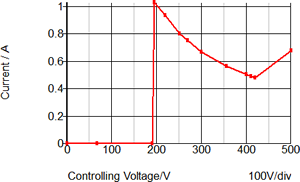# Handout E: SIMPLIS VPWL and IPWL Resistors

SIMPLIS has two PWL resistor models:

• VPWL: The resistor current is controlled by the terminal voltage
• IPWL: The resistor voltage is controlled by the resistor current

To decide which one to use, examine the PWL definition and determine if the Current is a function of Voltage or is the Voltage a function of Current. An example of a VPWL resistor is a constant power load. For each controlling Voltage, there exists one Current value. An example of a IPWL resistor is a ESD diode which models snapback. In this model, for each controlling Current there is one Voltage. These examples are included as a schematic 1.16_vpwl_ipwl_resistors.sxsch.

 Constant Power Load - VPWL ESD Diode with Snapback - IPWL